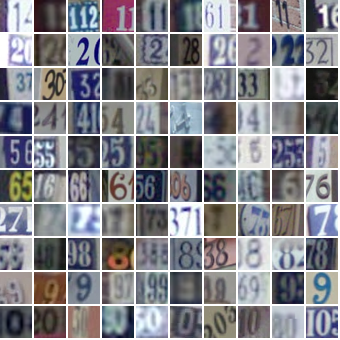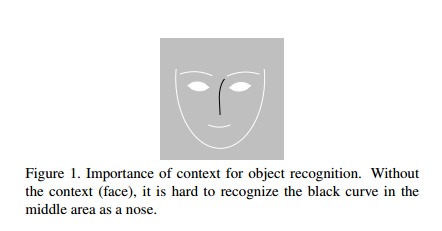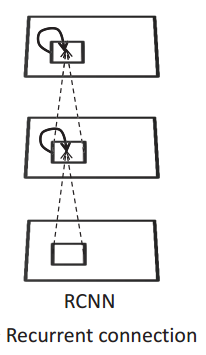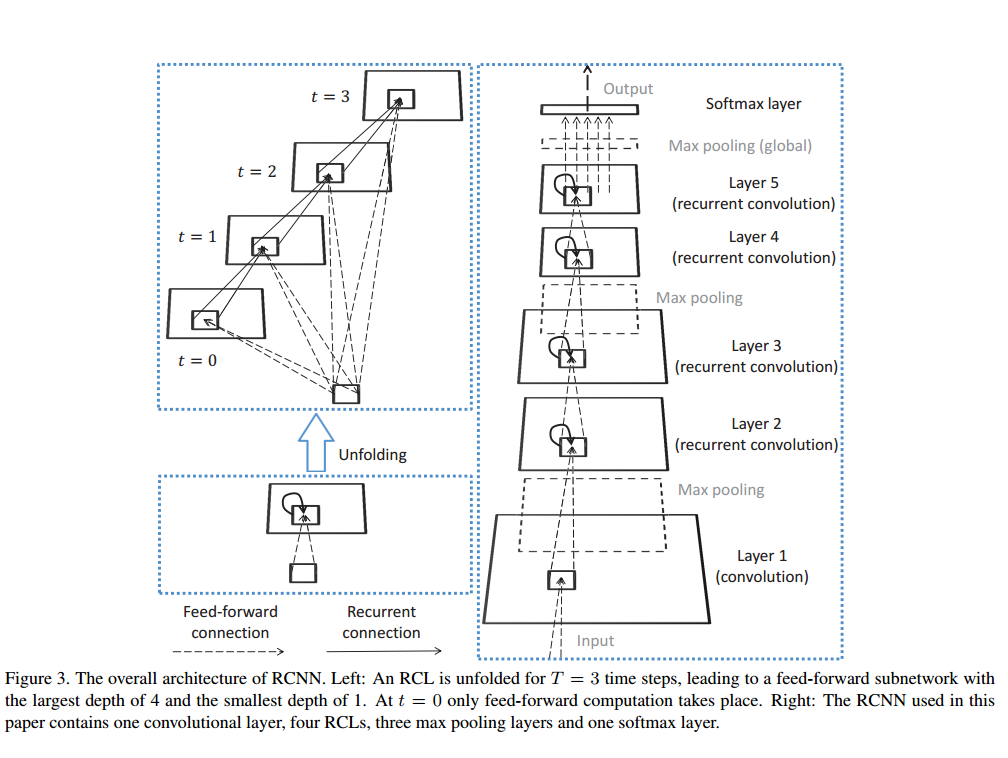# Recurrent Convolutional Neural Network For Svhn

2017-09-06-Recurren-Convolutional-Neural-Network-for-SVHN

## SVHN

Street View House Numbers (SVHN)是一个用于开发机器学习和对象识别算法的真实世界的图像数据集，对数据预处理和格式化的需求最小。它与MNIST相似(例如:图像是小的裁剪的数字)，但是包含了一个更大的标记的数据(超过60万的数字图像)，并且来自一个更加困难的、未解决的、真实的世界问题(在自然场景图像中识别数字和数字)。它是通过谷歌街景图片中的房屋号码获得的。## Recurrent Convolutional Neural Network(RCNN)

### 简介### Recurrent Convoluntional 层

Recurrent Convoluntional层(RCL)是RCNN的关键模块。RCL单元的的状态(state)会随着时间变化。在第k层feature map的位置(i, j)对应的特征公式为：

$z_{ijk}(t) = (w_k^f)^Tu^{(i,j)}(t) + (w_k^\gamma)^Tx^{(i,j)}(t-1) + b_k$ $(1)$

$x_{ijk}(t) = g(ReLU(z_{ijk}(t))$ $(2)$

$g(ReLU_{ijk}(t)) = \frac{ReLU_{ijk}(t)}{(1+\frac{\alpha}{N}\sum^{min(K, k+N/2)}_{k'=max(0, k-N/2)}(ReLU_{ijk'})^2)^\beta}$ $(3)$

K为feature maps的数量, $\alpha$$\beta$为常量控制归一化的振幅。### 整体结构

RCNN由多个RCL堆叠而成，可以选择在其中插入Max pooling层。整个结构如图三右侧所示。为了节省计算量，第一层为标准的前馈式卷积层，其后跟着max pooling。在其上，连接着4个RCL模块，并在中间插入max pooling层。两个RCL模块之间是前馈式连接。论文中，作者提供的max pooling的stride和size分别为2和3。最后RCNN结构并没有采用传统的全连接层用于输出，而是使用的全局式的max pooling对每一个feature map输出一个最大值用于表示图片。

## Code

Projecthttps://github.com/JimLee4530/RCNN

## 参考

 Liang, M., Hu, X.: Recurrent convolutional neural network for object recognition. In: Proceedings of the IEEE Conference on Computer Vision and Pattern Recognition

Written on September 6, 2017